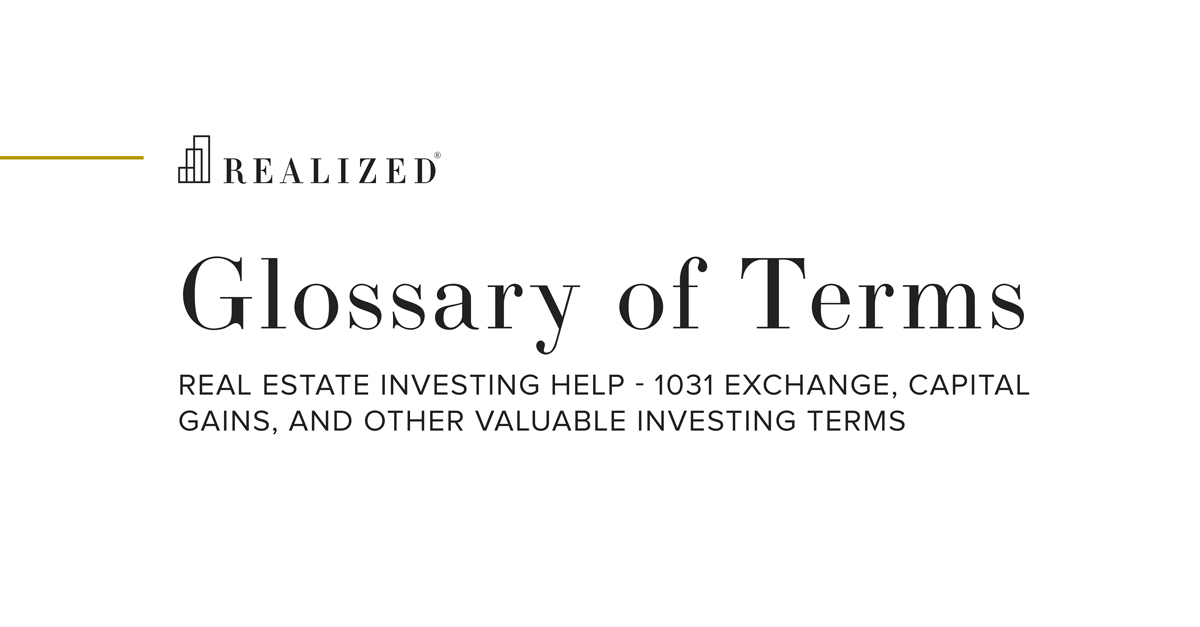Terminal Cap Rate 2020-10-09 08:00:00

The estimated or actual cap rate of a property on the date of disposition or sale. Also known as the exit cap rate and the reversionary cap rate. The terminal cap rate is a metric used to estimate an investment property's gross value at the sale (i.e., end of the holding period).

The terminal cap rate is also an important metric in determining the resale price of a property. The resale price is used for determining potential capital gains and the return on the property.

It is calculated by dividing the expected net operating income (NOI) by the expected sale price and is expressed as a percentage. For example, if the NOI in the year of sale (or the following year) is \$450,000 and the expected sale price is \$7,000,000, then the terminal cap rate would be 6.43% (NOI of \$450,000 divided by \$7,000,000 sale price).
In practice, the terminal cap rate is more typically applied to the estimated NOI in order to estimate terminal value.

Using a similar example, if an investor applied a 6.5% terminal cap rate to an estimated NOI of \$450,000, then the projected terminal value would be \$6,923,077 (NOI of \$450,000 divided by the terminal cap rate of 6.5%).

The formulas used in the previous paragraph are based on the direct income capitalization approach. When using this approach, a lower terminal cap rate means a higher resale value. There is an indirect relation between the terminal cap rate and sale value. As an example, if the above cap rate was 6%, the resale would be \$450,000/.06 = \$7.5 million. If the cap rate increases, the sale value will fall.